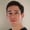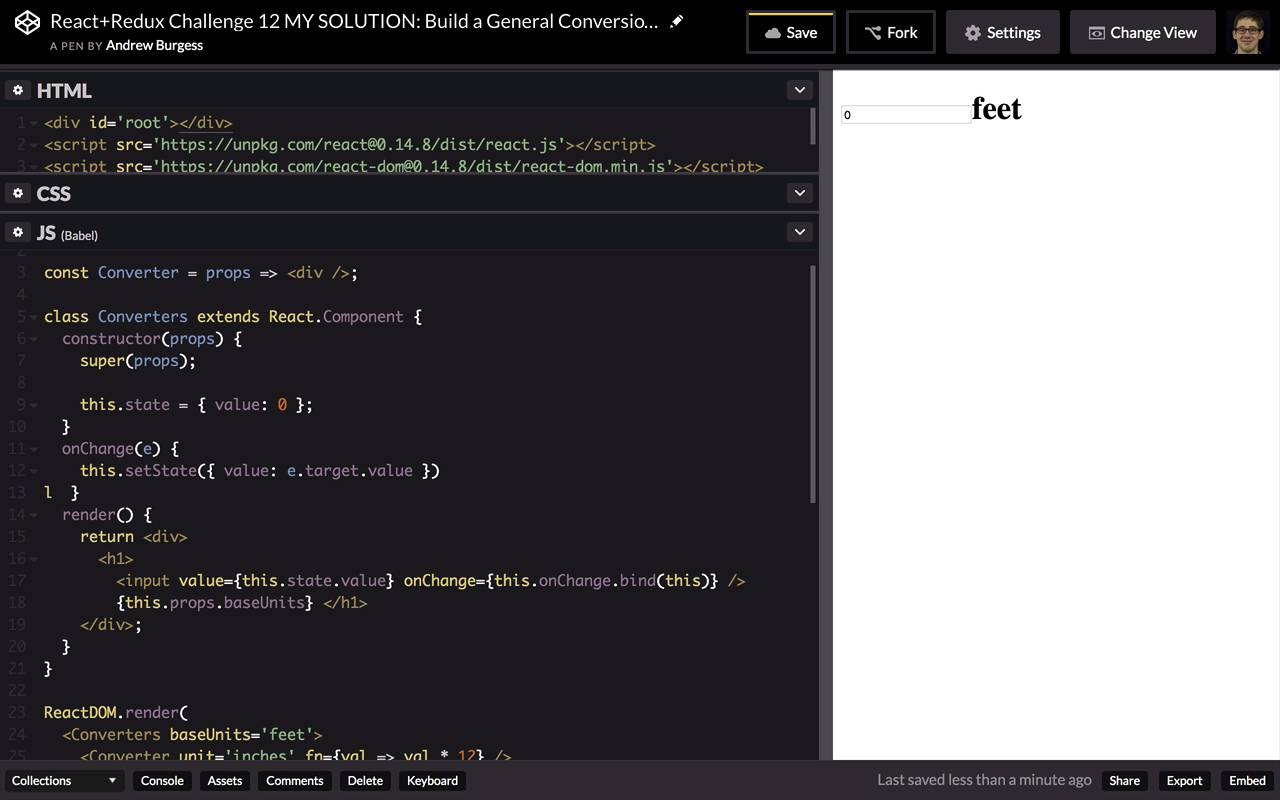FREELessons: 35Length: 4.1 hours• Overview
• Transcript

# 5.8 Challenge: General Conversion Component

This is a tricky one: your challenge is to build a <Converters> component that will have <Converter /> components inside it. You’ll see the API in the challenge—give it your best shot!

## 6.Conclusion1 lesson, 01:32

### 5.8 Challenge: General Conversion Component

This challenge, and the next challenge are going to be a little bit more challenging than what we've done previously. In fact, this one is a combination of a couple of things because there's quite a bit to build, but you may also have to do a little bit of research for this. In fact, there's a technique you might need to use in this challenge that is not mentioned at all anywhere else in this course. All right, the goal of this challenge is to build a general conversion component. So the idea here is that we have a converters component here which takes the base units and that in our case is going to be feet. And then what you can do is converter child components. They have a unit just for labeling and then they have a function which takes your value from the converters component and it will return whatever the number should be in the converted units. So for example here, if we get the number of feet passed in as the value here, we multiply that by 12 and we have inches. Just some basic arithmetic there. And so, it doesn't look too complicated, maybe on the surface. But remember that we need some way to pass a value from this converters function down to the converter child component. But we don't have a proper function to do that shown here, so you'll have to figure out how to do that. So if you would like to do this challenge yourself, go ahead and fork this. And I am going to show you now what my solution is. So let's begin with our converters component. So we have a converters component here. And this is going to be React.createClass. You can use ES class in text if you want. In fact, I easily read somewhere that it's possible in the future that React.createClass is going to be deprecated. And eventually I guess removed so maybe you wanna use ES6 class syntax. In fact you know what, let's do that. So we have class Converters extends React.Component. Let's get our render function going here. We'll just have a , and in here, at the top, we can start with an . And this will have an input in it. This will have a value which is gonna be this.state dot whatever the current value is. We'll have an onChange here in a second and then we'll have this.props.baseUnits printed after that. Okay, next we're going to need some initial state. When we use the class syntax, I guess we use the constructor method instead of get initial state. So we'll pass that to props and then we need to make sure that we pass the props to the super constructor. That's the constructor of React.Component. That's important. And then we can say this.state = and we'll set the value equal to 0, for starters. Okay, so looking good so far. We need an on change handler here, and the reason nothing's rendering yet is cuz we don't have our converter component. So maybe let's just throw that up here. This is gonna be simple. We'll say converter component is just gonna take some props in for now. We'll just return an empty div. So now you can see we are getting a little bit rendered here. We have 0 feet. But of course we can't change what's in this text box yet. So we need to add an on change handler to our input box here. So let's do this. Let's break this under a couple of lines and we can say onChange and we could actually do this in line but now let's just say this.onChange. And we'll have to bind that to this because in a class syntax, things are not bound automatically the way they are when we're using React.createClass. Okay, so up here we have onChange. This is gonna take an event. And this will be pretty simple. We'll do this.setState. And we will set the value to be e.target.value. Whatever is printed in the text box. So very basic but now we can actually put numbers in our text box. That's the easy part, really, that we've done so far. The next step is to actually render our converter components here. And the trick is that we need to somehow pass these converters an extra property that they aren't currently receiving. And we can kind of see why this is the case if we just pretend that they already receive a value property. So let's destructure this here. And we can say that they receive a unit and a value and the function, right? Yes. So we don't actually receive a value property but let's pretend that we do for now. And then what we can return here is I guess we'll just make this a paragraph and in here we can call the function, passing at the value. That's pretty straightforward, then we can just print the unit name after that, right? And we don't even need this on two lines, this is really simple. So that's really all we need to do. We need to pass the value of the function, and then we can label it with the unit. Okay, so let's see what happens now if underneath this, we go ahead and map over that. And why don't we put this in a list? So we'll change this paragraph up here to a list item tag. Then down here, we can put this in unordered list. And then inside our unordered list, we can do this.props.children. And we can map over each child. And ideally we would just actually render the child. It's because the child is already a React component, right? We wouldn't even need to map. We would just insert this.props.children like that, we would take this whole part out. But when we do that, we just get not a number inches, not a number centimeters and not a number miles. Because we don't actually have a value that we're passing to our function here. So instead we need to map over and somehow give this an extra property. And the way we can do this is by using the React method, React.cloneElement. The way this method works is we pass it an element. So for example, we pass it a child, each one of these children, but then, as a second property, we can pass it an object with some props. And these are extra props that we want to pass to our newly cloned element. So for example, we can have a key. And this key, we should probably take index, maybe, here as another parameter. So we have the key equals i, and then we can have the value equals this.state.value. And now as you can see, we actually have 0 centimeters, 0 inches, 0 miles showing up. So it looks like this might be working, let's see. If we change this to 1 foot, we get 12 inches, 30.48 centimeters and a very small bit of a mile. If we change this to 5,280, you can see we have 1 mile, a lot of inches and a lot of centimeters. So this is our generalized conversion component. It's kind of neat that we can use React.cloneElement in this way. Because what this allows us to do is create an API, for maybe a React utility library or something like that, that's nice and clean. The user doesn't have to worry about how these values are being passed around. It just provides a label and a function for converting. And then we have our conversion happening. So when I first found this, I thought this seemed a little bit hacky and I wondered if it was a best practice. But doing some research, I didn't find anyone who said that this was a bad practice. Of course I haven't really tested any of the performance impacts of doing this kind of thing. But if you have a simple application using React.cloneElement, might be a really neat way to create a better looking API for your JSX. So, that was our build a general conversion component challenge. If you were able to figure this out on your own, excellent work. Otherwise, I hope you learned something new and had some fun.

Back to the top